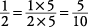## Renaming Fractions

The following section reviews the processes of renaming and simplifying fractions. These processes are invaluable when you add, subtract, multiply, or divide fractions.

#### Equivalent fractions

Fractions that name the same number, such as 1/2, 2/4, 3/6, 4/8, and 5/10, are called equivalent fractions. A simple method to check if fractions are equivalent is to cross multiply and check the products.

##### Example 1

Isequivalent to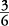?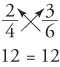The cross products are the same, so the fractionsandare equivalent.

##### Example 2

Is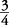equivalent to?The cross products are not the same, so the fractionsand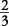are not equivalent.

#### Simplifying fractions

When given as a final answer, a fraction should be simplified to lowest terms. Simplifying fractions is done by dividing both the numerator and denominator by the largest integer that will divide evenly into both.

##### Example 3

Simplify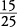to lowest terms.

To simplify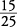to lowest terms, divide the numerator and denominator by 5.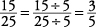Sincecannot be simplified any further, that is, the numerator and denominator cannot both be evenly divided again,is simplified to lowest terms.

##### Example 4

Simplifyto lowest terms.#### Enlarging denominators

The denominator of a fraction may be enlarged and the fraction keeps its original value by multiplying both the numerator and denominator by the same number.

##### Example 5

Changeto eighths.

To changeto eighths, simply multiply the numerator and denominator by 2.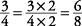##### Example 6

Express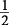as tenths.